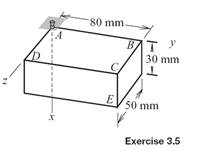### Create an Account

Already have account?

### Forgot Your Password ?

Home / Questions / Solve Exercise 35 if the rotations are θ1 65° about the Y axis followed by θ2 145° about ...

# Solve Exercise 35 if the rotations are θ1 65° about the Y axis followed by θ2 145° about the Z axis Exercise 35 The xyz coordinate system is attached to the box and XYZ is a parallel

Solve Exercise 3.5 if the rotations are θ= 65° about the Y axis, followed by θ2 = −145° about the Z axis.

Exercise 3.5

The xyz coordinate system is attached to the box, and XYZ is a parallel stationary coordinate system. The box undergoes a pair of rotations: First, θ1 = 65° about the y axis, followed by θ2 = −145° about the z axis. For this rotation determine (a) the coordinates relative to xyz in its final orientation of the stationary point that was at the location of point E prior to the rotations, (b) the coordinates relative to XYZ of corner E of the box after both rotations.Jun 18 2020 View more View Less

#### Answer (Solved)Subscribe To Get Solution